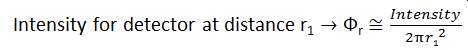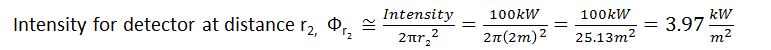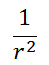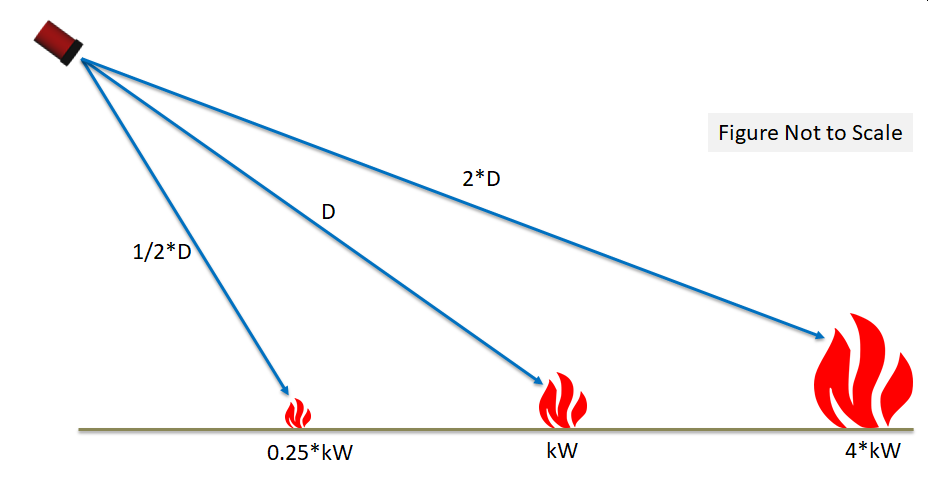# FOV Multiplier and Inverse Square Law Theory

The Field-Of-View (FOV) Multiplier is a factor which increase or decreases the maximum range of all detectors in the project. The factor can be from 0 to 10 and may have a decimal. A value of 1 indicates no change to the selected detector's FOV. A value less than one will reduce the maximum range, shown below.Tutorial 7 - Figure 1 - showing the FOV Multiplier reducing the maximum range of a detector

The FOV Multiplier is useful in conjunction with the Inverse Square Law in determining the maximum range of manufacturer provided FOV distances for varying fire sizes. You may use the Inverse Square Tool, described in the next section, when adding or editing the FOV Multiplier property of a risk grade. Note: The Inverse Square Law Calculator is not a replacement for manufacturer's data.

### Inverse Square Law Example:

To get an understanding of the inverse square law, let's first calculate the perceived intensity at a flame detector placed 1 meter away from a 100kW fire. The radiative power of the fire is theoretically distributed equally over the area of the dome and is calculated below.Tutorial 7 - Figure 2 - Diagram for calculating radiation intensity for a flame detector place 1 meter away from a 100kW fireKeeping this value in mind, let's now move the detector to be 2 meters from the same 100kW fire and calculate the intensity here.Tutorial 7 - Figure 3 - Diagram of updated intensity calculation when the detector is placed 2m away from the same 100kW fireAs the distance from the fire is increased, the intensity value reduces by, which is the inverse of the distance, squared - the basis for the inverse square law.

When considering ranges of flame detectors, if a device's maximum range was said to be 100ft for a tested fire of 40kw, then in order for the same device to "see" a 10kW fire it would have to be placed 50ft away. The opposite can also be applied, the same device could "see" a 160kW fire from 200ft away, as shown below. For mapping purposes, this would be used to perform analyses for fire sizes which are not specified by the manufacturer. It's important to understand that this is then making a mathematical correlation to tested data and users to be cautious when using this tool.Tutorial 7 - Figure 4 - Inverse Square Law relationship of flame detector distances to fire sizes. The middle example being the reference values